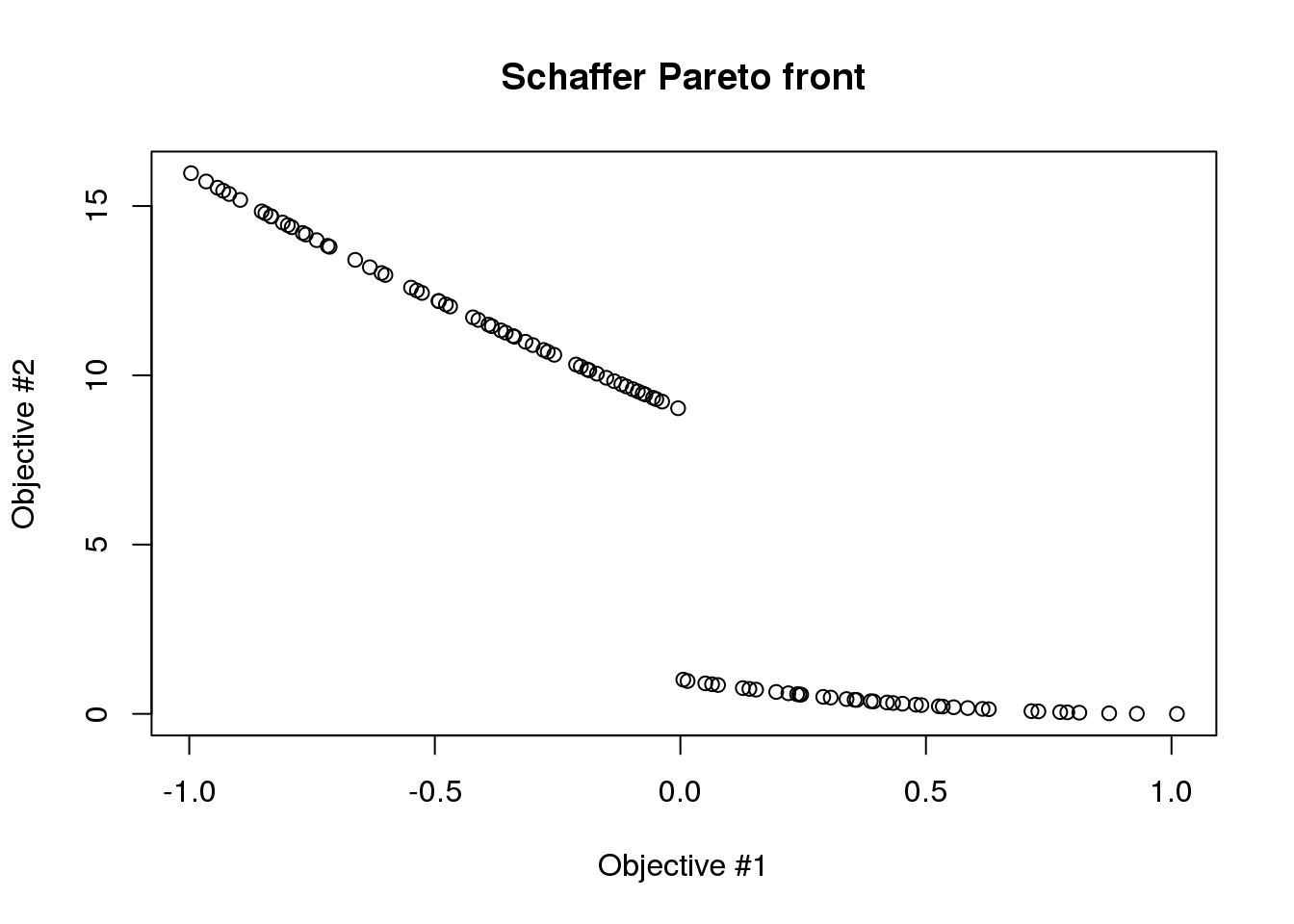# Short Description

caRamel is an R package for optimization implementing a multiobjective evolutionary algorithm combining the MEAS algorithm and the NGSA-II algorithm.

# Installation

`{r caRa} install.packages('caRamel')`

and then load it: `{r caRa} library(caRamel)`

# Test function

## Schaffer

Schaffer test function has two objectives with one variable.Schaffer test function

`{r schaffer} schaffer <- function(i) { if (x[i,1] <= 1) { s1 <- -x[i,1] } else if (x[i,1] <= 3) { s1 <- x[i,1] - 2 } else if (x[i,1] <= 4) { s1 <- 4 - x[i,1] } else { s1 <- x[i,1] - 4 } s2 <- (x[i,1] - 5) * (x[i,1] - 5) return(c(s1, s2)) }`

Note that :

• parameter i is mandatory for the management of parallelism.
• the variable must be named x and is a matrix of size [npopulation, nvariables].

The variable lies in the range [-5, 10]:

`{r schaffer_variable} nvar <- 1 # number of variables bounds <- matrix(data = 1, nrow = nvar, ncol = 2) # upper and lower bounds bounds[, 1] <- -5 * bounds[, 1] bounds[, 2] <- 10 * bounds[, 2]`

Both functions are to be minimized:

`{r schaffer_objectives} nobj <- 2 # number of objectives minmax <- c(FALSE, FALSE) # min and min`

Before calling caRamel in order to optimize the Schaffer’s problem, some algorithmic parameters need to be set:

`{r schaffer_param} popsize <- 100 # size of the genetic population archsize <- 100 # size of the archive for the Pareto front maxrun <- 1000 # maximum number of calls prec <- matrix(1.e-3, nrow = 1, ncol = nobj) # accuracy for the convergence phase`

Then the minimization problem can be launched:

`{r schaffer_launch, fig.show="hide", results="hide"} results <- caRamel(nobj, nvar, minmax, bounds, schaffer, popsize, archsize, maxrun, prec, carallel=FALSE) # no parallelism`

Test if the convergence is successful:

`{r schaffer_OK} print(results\$success==TRUE)`

Plot the Pareto front:

`{r schaffer_plot1} plot(results\$objectives[,1], results\$objectives[,2], main="Schaffer Pareto front", xlab="Objective #1", ylab="Objective #2")`

`{r schaffer_plot2} plot(results\$parameters, main="Corresponding values for X", xlab="Element of the archive", ylab="X Variable")`

• Efstratiadis, A. and Koutsoyiannis, D., The multiobjective evolutionary annealing-simplex method and its application in calibrating hydrological models, EGU General Assembly 2005, Geophysical Research Abstracts, vol.7, Vienna, European Geophysical Union
• Reed, P. and Devireddy, D., Groundwater monitoring design: a case study combining epsilon-dominance archiving and automatic parameterization for the NGSA-II, Coello-Coello C editor, Applications of multiobjective evolutionary algorithms, Advances in natural computation series, vol. 1, pp. 79-100, Word Scientific, New-York, 2004